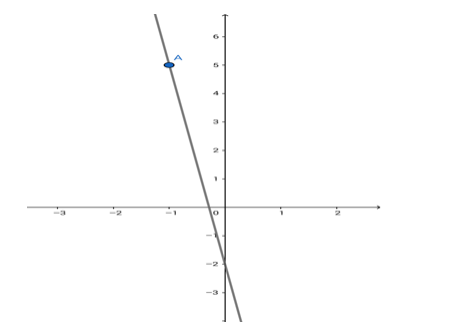# Find the equation of the line through the point

Question:

Find the equation of the line through the point ( - 1, 5) and making an intercept of - 2 on the y - axis.

Solution:Given: The $y$ - intercept $=-2$.

The line passes through $(-1,5)$.

Formula to be used: y = mx + c where m is the slope of the line and c is the y - intercept.

The equation of the line is $y=m x+(-2)=m x-2$.

Now, this line passes through $(-1,5)$.

$\therefore 5=m(-1)-2=-m-2$ i.e. $m=-(5+2)=-7$

$\therefore y=(-7) x+(-2)=-7 x-2$ i.e. $7 x+y+2=0$